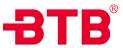• 系列号
L=直角阀
• 功能
7=常闭
8=常开
9=双作用
10=自由态
• 阀体材质
1=316L
2=316
3=304
• 执行器材质
4=铝合金
5=304不锈钢
• 主要密封材质
9=PTFE(-20℃ ~ +180℃)
1=PTFE复合(-20℃ ~ +220℃)
• 执行器尺寸
05=50mm
06=63mm
08=80mm
• 接口
G=英制直管螺纹BSP DIN ISO228-1(国内G)
T=英制锥管螺纹BSPT DIN2999-1(国内Rc)
N=美制锥管螺纹NPTASMEB1.20.1
K=快装式ISO2852
K2=快装式定制尺寸
F=法兰式HG5010-58
F2=法兰式定制尺寸
• 规格
-15=1/2"(DN15)
-20=3/4"(DN20)
-25=1"(DN25)
-32=1 1/4"（DN32）
-40=1 1/2" (DN40)
• 附件
-F1=智能定位器
-F2=新型智能定位器
-F3=两位三通电磁阀
-F4=两位五通电磁阀
-F5=双位反馈器
-F6=线式信号反馈器：a-高频 b-低频
-F9=气源调压过滤器